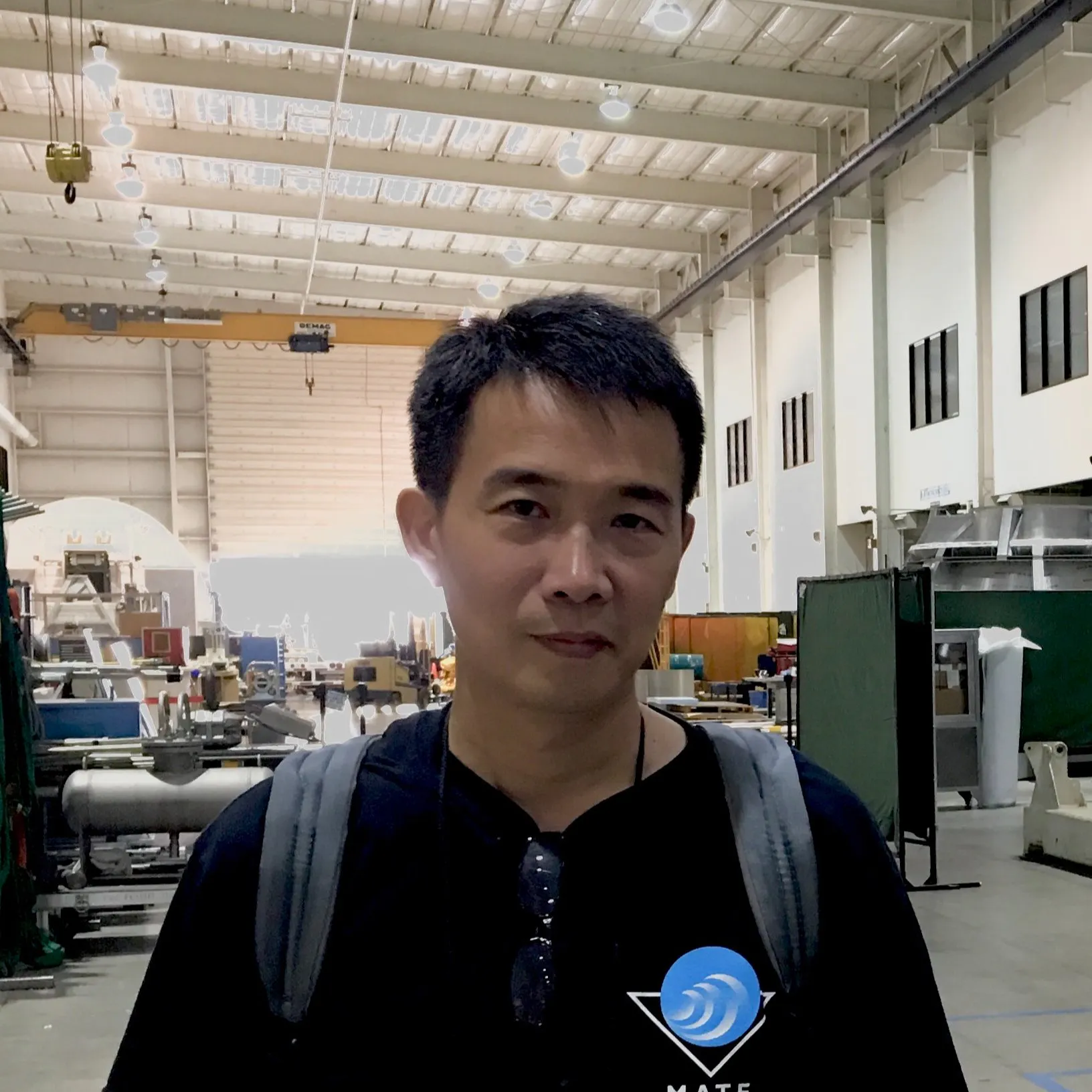# AR Pong Game with Object Detection

The ML model uses Edge Impulse's FOMO (Faster Objects, More Objects) to detect and differentiate which players are playing & the coordinates

IntermediateFull instructions provided8 hours250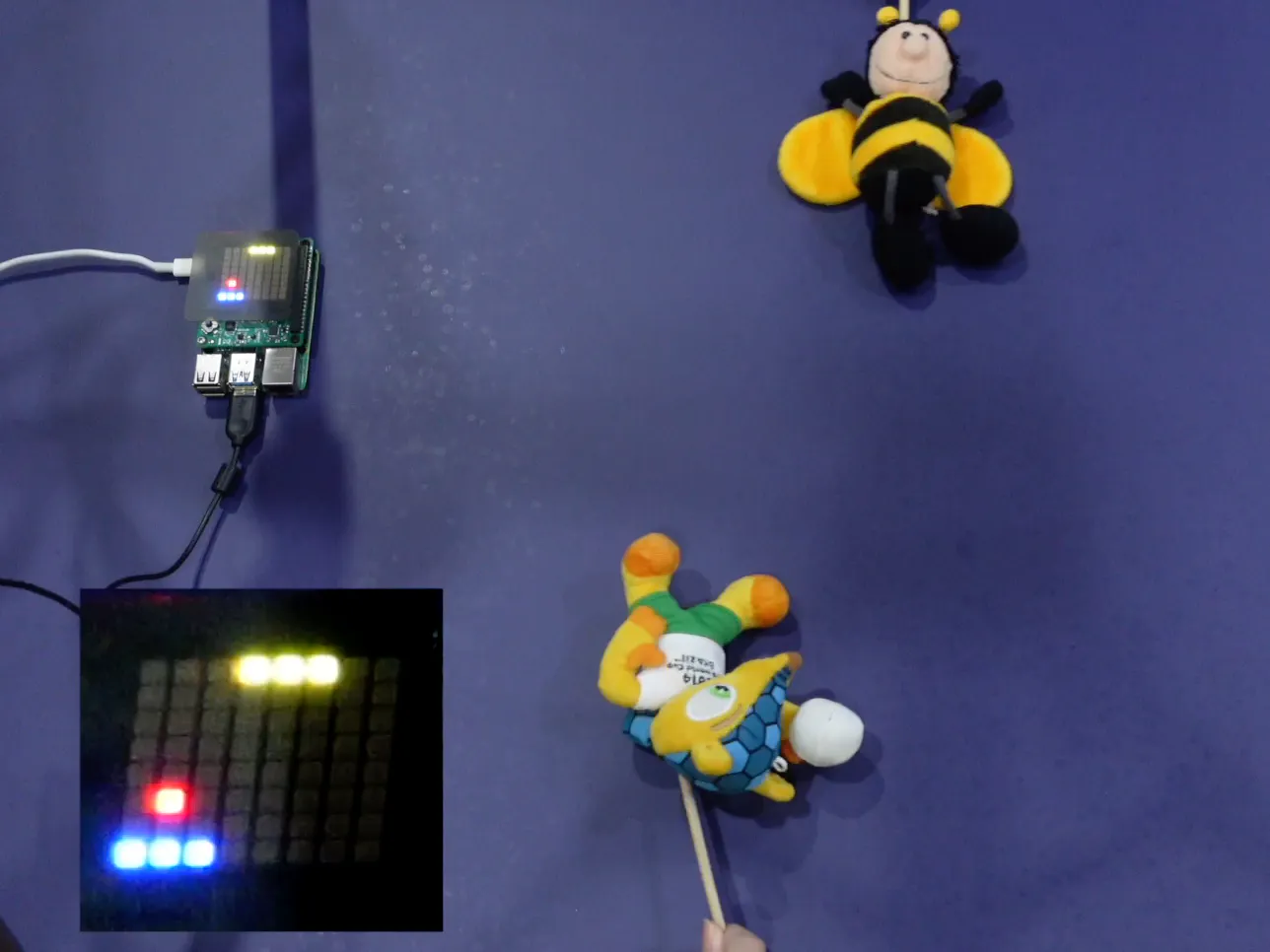## Things used in this project

### Hardware components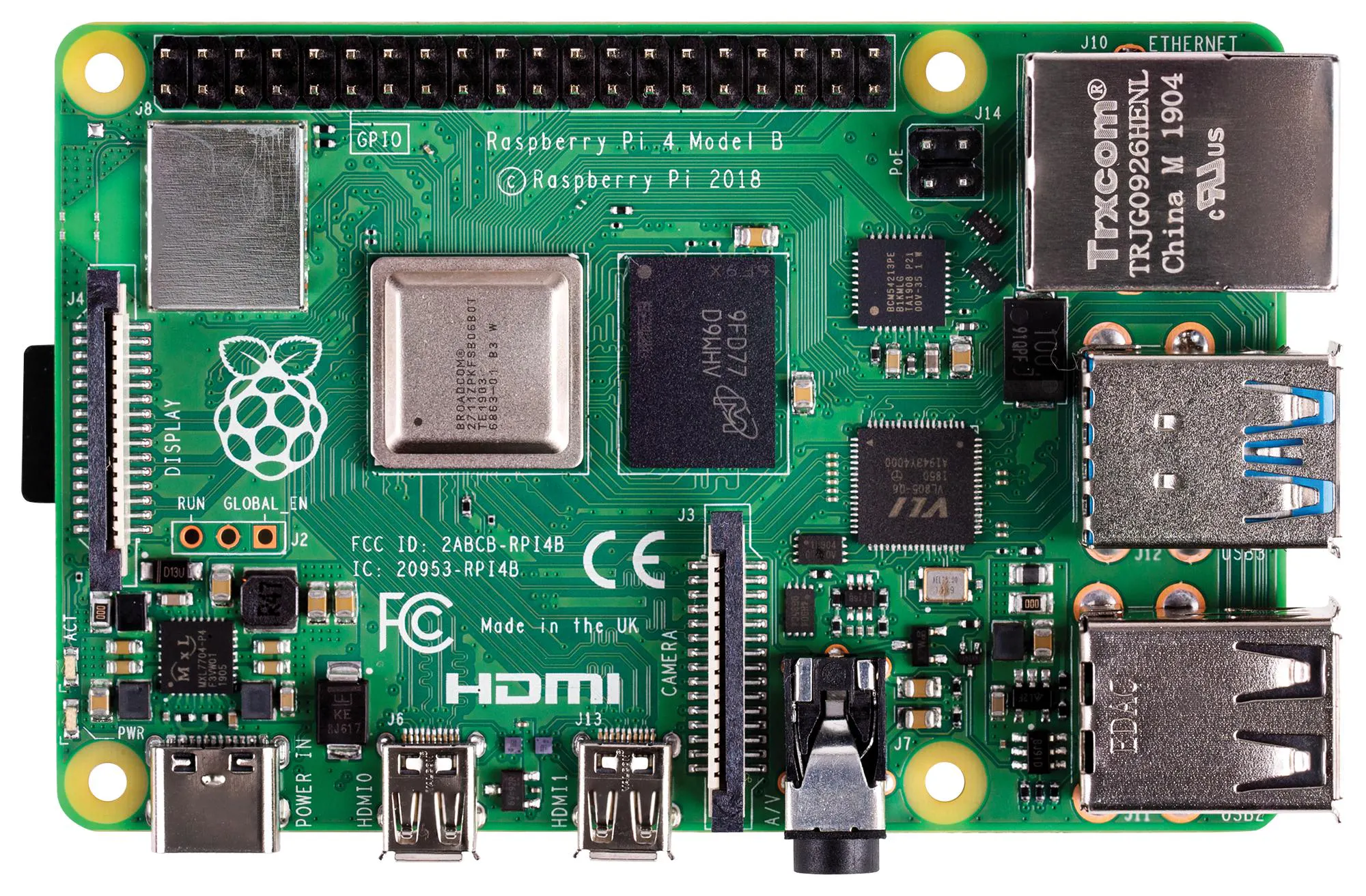Raspberry Pi 4 Model B
×1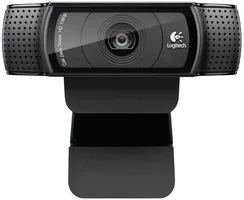Webcam, Logitech® HD Pro Logitech C270 or other USB Webcam
×1
 Animal plush toys Or whatever objects to detect
×1
 Camera tripod
×1

### Software apps and online servicesEdge Impulse Studio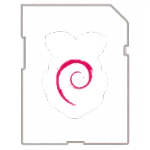Raspberry Pi Raspbian I use Debian version 10 (buster)
 Your favorite IDE eg. Sublime Text
 Terminal App

## Schematics

### Setup diagram

SenseHAT, Pi4, USB webcam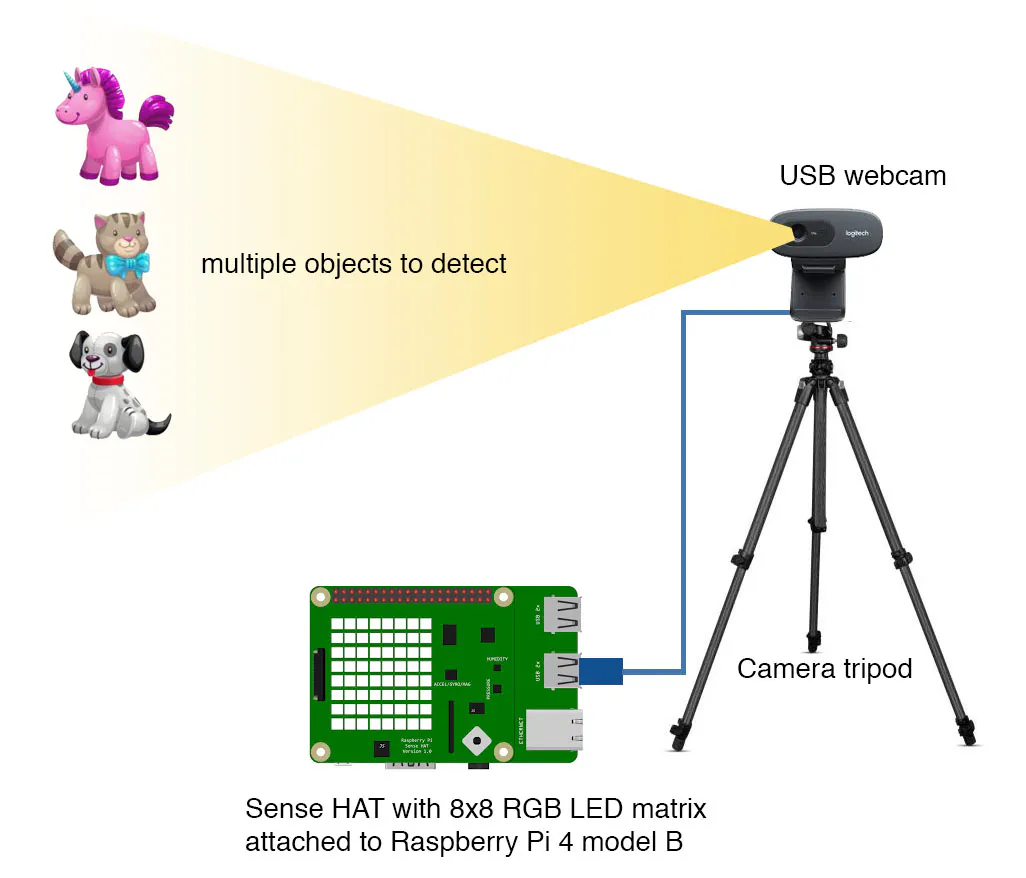## Code

### pong_1_objects.py

Python
This program can detect which object/player will play this game.
```#!/usr/bin/env python

#import device_patches       # Device specific patches for Jetson Nano (needs to be before importing cv2)

import cv2
import os
import sys, getopt
import signal
import time
from edge_impulse_linux.image import ImageImpulseRunner
from sense_hat import SenseHat
from time import sleep

sense = SenseHat()

# class and objects to show the players

class Player:
def __init__(self, colours, picture):
self.picture = [[colours[ord(id) - 48] for id in row] for row in picture]
self.height = len(self.picture)
self.width = len(self.picture)
self.y_move = self.height - 8
self.x_move = self.width - 8
self.frames = (self.y_move + self.x_move) * 2

def draw(self, frame):
frame %= self.frames
xShift = frame if frame < self.x_move else\
self.x_move if frame < self.x_move + self.y_move else\
self.x_move * 2 + self.y_move - frame if frame < self.x_move * 2 + self.y_move else\
0
yShift = 0 if frame < self.x_move else\
frame - self.x_move if frame < self.x_move + self.y_move else\
self.y_move if frame < self.x_move * 2 + self.y_move else\
self.x_move * 2 + self.y_move * 2 - frame
to_set = []
for i in range(yShift, yShift + 8):
to_set += self.picture[i][xShift:xShift + 8]
sense.set_pixels(to_set)

def animate(self, duration):
frame = 0
duration += time.time()
while time.time() < duration:
self.draw(frame)
frame += 1
sleep(min(duration - time.time(), .15))

def mid(self, x_, y_):
to_set = []
for i in range(y_, y_ + 8):
to_set += self.picture[i][x_:x_ + 8]
sense.set_pixels(to_set)

bee = Player([
(200, 200, 0), # 0 = yellow
(0, 0, 200), # 1 = blue
(200, 200, 200), # 2 = white
(0, 0, 0)], # 3 = black
["11133333111",
"11322322311",
"11132232311",
"11113333311",
"11133030031",
"11303030003",
"33303030303",
"11303030003",
"11133030031",
"11113333311",
"11111313111"])

arma = Player([
(0, 0, 200), # 0 = blue
(200, 0, 0), # 1 = red
(200, 200, 200), # 2 = white
(200, 140, 0), # 3 = orange
(0, 0, 0), # 4 = black
(60, 40, 20)], # 5 = chocolate
["55555555535",
"55555555335",
"55555553133",
"55555503113",
"55555003130",
"55500033333",
"50003332233",
"11333324223",
"13333332233",
"55333333333",
"55554433335"])

runner = None
# if you don't want to see a camera preview, set this to False
show_camera = True
if (sys.platform == 'linux' and not os.environ.get('DISPLAY')):
show_camera = False

def now():
return round(time.time() * 1000)

def get_webcams():
port_ids = []
for port in range(5):
print("Looking for a camera in port %s:" %port)
camera = cv2.VideoCapture(port)
if camera.isOpened():
if ret:
backendName = camera.getBackendName()
w = camera.get(3)
h = camera.get(4)
print("Camera %s (%s x %s) found in port %s " %(backendName,h,w, port))
port_ids.append(port)
camera.release()
return port_ids

def sigint_handler(sig, frame):
print('Interrupted')
if (runner):
runner.stop()
sys.exit(0)

signal.signal(signal.SIGINT, sigint_handler)

def help():
print('python classify.py <path_to_model.eim> <Camera port ID, only required when more than 1 camera is present>')

ball_position=[6, 3]
ball_velocity=[-1, -1]
player_position = 4

def draw_bat(col):
r, g, b = col
sense.set_pixel(0, player_position-1, r, g, b)
sense.set_pixel(0, player_position, r, g, b)
sense.set_pixel(0, player_position+1, r, g, b)

ball_move_delay = 250 # number of milliseconds before the ball moves

def ball_play():
global next_time_to_move
if next_time_to_move == 0:
next_time_to_move = now()
if next_time_to_move <= now():
next_time_to_move = next_time_to_move + ball_move_delay
ball_position += ball_velocity
ball_position += ball_velocity

if ball_position == 0 or ball_position == 7:
ball_velocity = -ball_velocity
if ball_position == 7:
ball_velocity = -ball_velocity
if ball_position == 1 and\
player_position-1 <= ball_position <= player_position+1:
ball_velocity = -ball_velocity
if ball_position == 0:
return False

sense.set_pixel(ball_position, ball_position, 255, 0, 0)
return True

def main(argv):
try:
opts, args = getopt.getopt(argv, "h", ["--help"])
except getopt.GetoptError:
help()
sys.exit(2)

for opt, arg in opts:
if opt in ('-h', '--help'):
help()
sys.exit()

if len(args) == 0:
help()
sys.exit(2)

model = args

dir_path = os.path.dirname(os.path.realpath(__file__))
modelfile = os.path.join(dir_path, model)

print('MODEL: ' + modelfile)

with ImageImpulseRunner(modelfile) as runner:
try:
model_info = runner.init()
print('Loaded runner for "' + model_info['project']['owner'] + ' / ' + model_info['project']['name'] + '"')
labels = model_info['model_parameters']['labels']
if len(args)>= 2:
videoCaptureDeviceId = int(args)
else:
port_ids = get_webcams()
if len(port_ids) == 0:
raise Exception('Cannot find any webcams')
if len(args)<= 1 and len(port_ids)> 1:
raise Exception("Multiple cameras found. Add the camera port ID as a second argument to use to this script")
videoCaptureDeviceId = int(port_ids)

camera = cv2.VideoCapture(videoCaptureDeviceId)
if ret:
backendName = camera.getBackendName()
w = camera.get(3)
h = camera.get(4)
print("Camera %s (%s x %s) in port %s selected." %(backendName,h,w, videoCaptureDeviceId))
camera.release()
else:
raise Exception("Couldn't initialize selected camera.")

global next_time_to_move
next_time_to_move = 0
player = None

for res, img in runner.classifier(videoCaptureDeviceId):

sense.clear(0, 0, 0)

batCol = (255, 255, 0)
# optionally colour the bat white if the object is not detected
# batCol = (255, 255, 255)

if "bounding_boxes" in res["result"].keys():
for bb in res["result"]["bounding_boxes"]:
if player == None:
player = bb['label']
if player == 'bee':
bee.animate(3.6)
elif player == 'arma':
arma.animate(3.6)
if bb['label'] == player:
global player_position
player_position = min(max(bb['y'] // 8 - 1, 1), 6)
batCol = (255, 255, 0)

if player == None:
continue

cont = ball_play()
if not cont: break
draw_bat(batCol)

sense.show_message("Game Over", text_colour=(255, 0, 0))

finally:
if (runner):
runner.stop()

if __name__ == "__main__":
main(sys.argv[1:])
```

### pong_2_objects.py

Python
```#!/usr/bin/env python

#import device_patches       # Device specific patches for Jetson Nano (needs to be before importing cv2)

import cv2
import os
import sys, getopt
import signal
import time
from edge_impulse_linux.image import ImageImpulseRunner
from sense_hat import SenseHat
from time import sleep

sense = SenseHat()

# class and objects to show the players

class Player:
def __init__(self, colours, picture):
self.picture = [[colours[ord(id) - 48] for id in row] for row in picture]
self.height = len(self.picture)
self.width = len(self.picture)
self.y_move = self.height - 8
self.x_move = self.width - 8
self.frames = (self.y_move + self.x_move) * 2

def draw(self, frame):
frame %= self.frames
xShift = frame if frame < self.x_move else\
self.x_move if frame < self.x_move + self.y_move else\
self.x_move * 2 + self.y_move - frame if frame < self.x_move * 2 + self.y_move else\
0
yShift = 0 if frame < self.x_move else\
frame - self.x_move if frame < self.x_move + self.y_move else\
self.y_move if frame < self.x_move * 2 + self.y_move else\
self.x_move * 2 + self.y_move * 2 - frame
to_set = []
for i in range(yShift, yShift + 8):
to_set += self.picture[i][xShift:xShift + 8]
sense.set_pixels(to_set)

def animate(self, duration):
frame = 0
duration += time.time()
while time.time() < duration:
self.draw(frame)
frame += 1
sleep(min(duration - time.time(), .15))

def mid(self, x_, y_):
to_set = []
for i in range(y_, y_ + 8):
to_set += self.picture[i][x_:x_ + 8]
sense.set_pixels(to_set)

bee = Player([
(200, 200, 0), # 0 = yellow
(0, 0, 200), # 1 = blue
(200, 200, 200), # 2 = white
(0, 0, 0)], # 3 = black
["11133333111",
"11322322311",
"11132232311",
"11113333311",
"11133030031",
"11303030003",
"33303030303",
"11303030003",
"11133030031",
"11113333311",
"11111313111"])

arma = Player([
(0, 0, 200), # 0 = blue
(200, 0, 0), # 1 = red
(200, 200, 200), # 2 = white
(200, 140, 0), # 3 = orange
(0, 0, 0), # 4 = black
(60, 40, 20)], # 5 = chocolate
["55555555535",
"55555555335",
"55555553133",
"55555503113",
"55555003130",
"55500033333",
"50003332233",
"11333324223",
"13333332233",
"55333333333",
"55554433335"])

runner = None
# if you don't want to see a camera preview, set this to False
show_camera = True
if (sys.platform == 'linux' and not os.environ.get('DISPLAY')):
show_camera = False

def now():
return round(time.time() * 1000)

def get_webcams():
port_ids = []
for port in range(5):
print("Looking for a camera in port %s:" %port)
camera = cv2.VideoCapture(port)
if camera.isOpened():
if ret:
backendName = camera.getBackendName()
w = camera.get(3)
h = camera.get(4)
print("Camera %s (%s x %s) found in port %s " %(backendName,h,w, port))
port_ids.append(port)
camera.release()
return port_ids

def sigint_handler(sig, frame):
print('Interrupted')
if (runner):
runner.stop()
sys.exit(0)

signal.signal(signal.SIGINT, sigint_handler)

def help():
print('python classify.py <path_to_model.eim> <Camera port ID, only required when more than 1 camera is present>')

ball_position=[6, 3]
ball_velocity=[-1, -1]
bee_position = 4
arma_position = 4

def draw_bats():
sense.set_pixel(0, bee_position-1, 255, 255, 0)
sense.set_pixel(0, bee_position, 255, 255, 0)
sense.set_pixel(0, bee_position+1, 255, 255, 0)

sense.set_pixel(7, arma_position-1, 0, 0, 255)
sense.set_pixel(7, arma_position, 0, 0, 255)
sense.set_pixel(7, arma_position+1, 0, 0, 255)

ball_move_delay = 300

def ball_play():
global next_time_to_move
if next_time_to_move == 0:
next_time_to_move = now()
if next_time_to_move <= now():
next_time_to_move = next_time_to_move + ball_move_delay
ball_position += ball_velocity
ball_position += ball_velocity

if ball_position == 0 or ball_position == 7:
ball_velocity = -ball_velocity
if ball_position == 6 and\
arma_position-1 <= ball_position <= arma_position+1:
ball_velocity = -ball_velocity
if ball_position == 1 and\
bee_position-1 <= ball_position <= bee_position+1:
ball_velocity = -ball_velocity
if ball_position == 0:
return "arma"
if ball_position == 7:
return "bee"

sense.set_pixel(ball_position, ball_position, 255, 0, 0)
return "continue"

def main(argv):
try:
opts, args = getopt.getopt(argv, "h", ["--help"])
except getopt.GetoptError:
help()
sys.exit(2)

for opt, arg in opts:
if opt in ('-h', '--help'):
help()
sys.exit()

if len(args) == 0:
help()
sys.exit(2)

model = args

dir_path = os.path.dirname(os.path.realpath(__file__))
modelfile = os.path.join(dir_path, model)

print('MODEL: ' + modelfile)

with ImageImpulseRunner(modelfile) as runner:
try:
model_info = runner.init()
print('Loaded runner for "' + model_info['project']['owner'] + ' / ' + model_info['project']['name'] + '"')
labels = model_info['model_parameters']['labels']
if len(args)>= 2:
videoCaptureDeviceId = int(args)
else:
port_ids = get_webcams()
if len(port_ids) == 0:
raise Exception('Cannot find any webcams')
if len(args)<= 1 and len(port_ids)> 1:
raise Exception("Multiple cameras found. Add the camera port ID as a second argument to use to this script")
videoCaptureDeviceId = int(port_ids)

camera = cv2.VideoCapture(videoCaptureDeviceId)
if ret:
backendName = camera.getBackendName()
w = camera.get(3)
h = camera.get(4)
print("Camera %s (%s x %s) in port %s selected." %(backendName,h,w, videoCaptureDeviceId))
camera.release()
else:
raise Exception("Couldn't initialize selected camera.")

bee.animate(1.8)
arma.animate(1.8)
global next_time_to_move
next_time_to_move = 0

status = 0

for res, img in runner.classifier(videoCaptureDeviceId):

sense.clear(0, 0, 0)

if "bounding_boxes" in res["result"].keys():
for bb in res["result"]["bounding_boxes"]:
if bb['label'] == 'arma':
global arma_position
arma_position = min(max(bb['y'] // 8 - 1, 1), 6)
elif bb['label'] == 'bee':
global bee_position
bee_position = min(max(bb['y'] // 8 - 1, 1), 6)

status = ball_play()
if status != "continue": break
draw_bats()

if status == "arma":
arma.animate(3.6)
elif status == "bee":
bee.animate(3.6)

sense.show_message("Game Over", text_colour=(255, 0, 0))

finally:
if (runner):
runner.stop()

if __name__ == "__main__":
main(sys.argv[1:])
```

## Credits

### Jallson Suryo

3 projects • 15 followers
Tech integrator for schools. Also works as a maker and his activities include disassembling, fixing, and making things.
Thanks to Nicholas Patrick.